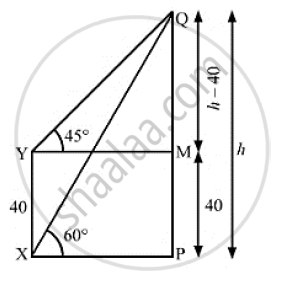# The Angle of Elevation of the Top Q of a Vertical Tower Pq from a Point X on the Ground is 60° . at a Point Y, 40m Vertically Above X, the Angle of Elevation is 45° . Find the Height of Tower Pq. - Mathematics

The angle of elevation of the top Q of a vertical tower PQ from a point X on the ground is 60° . At a point Y, 40m vertically above X, the angle of elevation is 45° . Find the height of tower PQ.

#### SolutionWe have
XY = 40m,∠PXQ = 60° and ∠MYQ = 45°
Let PQ = h
Also, MP = XY = 40m, MQ =  PQ  - MP = h - 40
In ΔMYQ,

 tan 45° = (MQ)/(MY)

⇒1 = (h-40)/(MY)

⇒ MY = H - 40

⇒ PX = MY = h - 40                       ................(1)

Now , in ΔMXQ,

tan 60° = (PQ)/(PX)

⇒ sqrt(3) = h/( h-40)                         [From (i)]

⇒ h sqrt(3) - 40 sqrt(3) = h

⇒ h sqrt(3) -h = 40 sqrt(3)

⇒ h  (sqrt(3)-1) = 40 sqrt(3)

⇒ h = (40sqrt(3))/((sqrt(3)-1))

⇒ h = (40 sqrt(3))/((sqrt (3)-1)) xx ((sqrt(3)+1))/((sqrt(3)+1))

⇒ h = (40 sqrt(3)(sqrt(3)+1))/((3-1))

⇒h = (40 sqrt(3) ( sqrt(3) +1))/2

⇒h= 20sqrt(3) (sqrt(3)+1)

⇒ h= 60+20 sqrt(3)

⇒ h= 60+20xx 1.73

⇒h = 60+ 34.6

∴ h = 94.6m

So, the height of the tower PQ is 94. 6 m.

Concept: Heights and Distances
Is there an error in this question or solution?
Chapter 14: Height and Distance - Exercises

#### APPEARS IN

RS Aggarwal Secondary School Class 10 Maths
Chapter 14 Height and Distance
Exercises | Q 24

Share